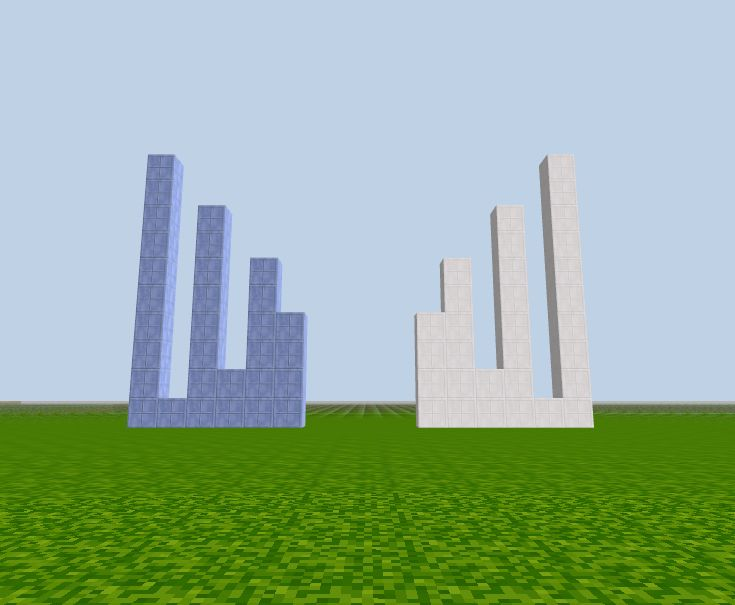# Array Methods

Array is one of the most useful data structures in software. There are many methods available to help manipulate data inside arrays. In this chapter we will cover a few frequently used methods.

### Add/remove elements at the end

`push()` adds an element to the end. `pop()` removes an element from the end and returns its value.

Examples:

``````let dates = ['Sun', 'Mon', 'Tue', 'Wed', 'Thu', 'Fri', 'Sat'];

// remove the last item
dates.pop();
console.log(dates);   // ['Sun', 'Mon', 'Tue', 'Wed', 'Thu', 'Fri']

// add an item at the end
dates.push(6);

// add another item at the end, using index
dates[dates.length] = 7;

console.log(dates);   // ['Sun', 'Mon', 'Tue', 'Wed', 'Thu', 'Fri', 6, 7]

// push can add multiple elements at once:
dates.push(8, 9, 10);
console.log(dates);   // ['Sun', 'Mon', 'Tue', 'Wed', 'Thu', 'Fri', 6, 7, 8, 9, 10]
``````

The call to `dates.push(6)` works the same as `dates[dates.length] = 6`. They both add an element at the end of the array. `push()` is better in this case because it makes the code easier to understand.

### Slice an array

The `slice()` method copies a piece of an array into a new array. The original array is not changed.

The `slice()` method can take two arguments in the form of `slice(start, end)`. The method then selects elements from the index `start`, and up to (but not including) the index `end`.

If there is only one argument, it's treated as `start`: `slice(start)`. Then slice goes from the `start` index to the end of the array.

Example:

``````let nums = [0, 1, 2, 3, 4, 5, 6, 7, 8];

let n1 = nums.slice(2, 5);
console.log(n1);       // [2, 3, 4]

let n2 = nums.slice(5);
console.log(n2);       // [5, 6, 7, 8]
``````

### Insert and remove elements

JavaScript array does not have `insert()` and `remove()` methods. Instead, it has one method that can do both: `splice()`.

The syntax:

`splice(index, deleteCount, items...)`

This method finds the element at `index`, deletes `deleteCount` elements then insert `items` at this place.

Examples:

``````let numbers = [0, 1, 2, 3, 4, 5, 6];
numbers.splice(2, 3);    // from index 2 remove 3 elements
console.log(numbers);    // [0, 1, 5, 6]

numbers.splice(1, 1, "one", "two", "three");      // from index 1 replace 1 element with some 3 string elements
console.log(numbers);     // [0, "one", "two", "three", 5, 6]

// no delete, just insert elements
numbers.splice(4, 0, "FOUR");
console.log(numbers);       // [0, "one", "two", "three", "FOUR", 5, 6]
``````

### Manipulate elements order

##### Reverse the order of all elements in array

The method `arr.reverse()` reverses the array in-place. It does not generate a new array.

``````let numbers = [1, 2, 3, 4, 5];
numbers.reverse();

console.log(numbers);     // 5,4,3,2,1
``````

### Convert between string and array

##### String to array

`split()` – converts a string to an array. It splits the string into an array by the given delimiter or seperator as in `str.split(delimiter)`.

In the example below, the delimiter is a comma followed by space:

``````let counts = 'One, Two, Three, Four';

let nums = counts.split(', ');

console.log(nums);
``````

Results:

``````["One", "Two", "Three", "Four"]
``````

Calling split(s) with an empty delimiter argument would split the string into an array of letters:

``````let str = "test";

console.log(str.split(''));      // ['t', 'e', 's', 't']
``````
##### Array to string

`arr.join(s)` does the opposite of `str.split()`. It connects the items of `arr` with `s` in between and creates a string.

Example:

``````let bestCs = ['chocolate', 'cake', 'candy'];

let str = bestCs.join('-');

console.log(str); // "chocolate-cake-candy"
``````
##### min() and max()

If you are a JavaScript beginner you can skip this section.

JavaScript has functions to get the minimum and maximum numbers in a list:

``````let min = Math.min(4, 6, 2, 9);
let max = Math.max(4, 6, 2, 9);

console.log(min, max);
``````

Either function can accept any number of arguments, but you cannot pass an array as argument. The following code does not give the right result:

``````let numbers = [4, 6, 2, 9];
let min = Math.min(numbers);

console.log(min);
``````

If you know the `numbers` array has exactly 4 elements, then you can use

``````Math.min(numbers, numbers, numbers, numbers);
``````

But that code doesn't look pretty, and most arrays don't have a small and fixed number of elements. JavaScript has a spread operator `...` that can unpack an array so that you can use the elements as function arguments easily.

``````let numbers = [4, 6, 2, 9];
let min = Math.min(...numbers);

console.log(min);
``````

The spread operator can be used in other places where a list of values is needed.

``````let numbers = [4, 6, 2, 9];
let copy = [...numbers];
``````

In this example, the `numbers` array is unpacked, then the values are used to create another array. Now `copy` contains exactly the same content. But they are different arrays so if you modify one array, the other does not change.

You can also use the spread operator to build an array from multiple arrays:

``````let numbers = [1, 2, 3];
let moreNumbers = [4, 5];
let allNumbers = [...numbers, ...moreNumbers];

console.log(allNumbers);  // [1, 2, 3, 4, 5]
``````

### CodeCraft visual

Let's use CodeCraft to demo some array methods:

``````// original array, white columns at the left
let t = [2, 4, 1, 8, 5, 6, 7]

for (let i in t) {
column_m(i - 10, -20, t[i], 15);       // box_white: 15
}

// pop(), last item popped out(red at far right)
column_m(t.length, -20, t.pop(), 13);

// new array at the right, yellow
for (let i in t) {
column_m(i, -20, t[i], 16);
}
``````The reverse() method reverses the elements in an array.

``````// original array, white
let num = [4, 6, 2, 8, 1, 10];
for (let i in num) {
column_m(i, -20, num[i], 15);       // white
}

// reverse the array
num.reverse();
for (let i in num) {
column_m(i-10, -20, num[i], 2);     // blue
}
``````

See in the picture, the blue columns mirror the white columns.